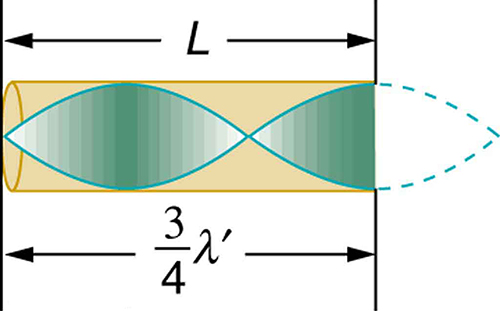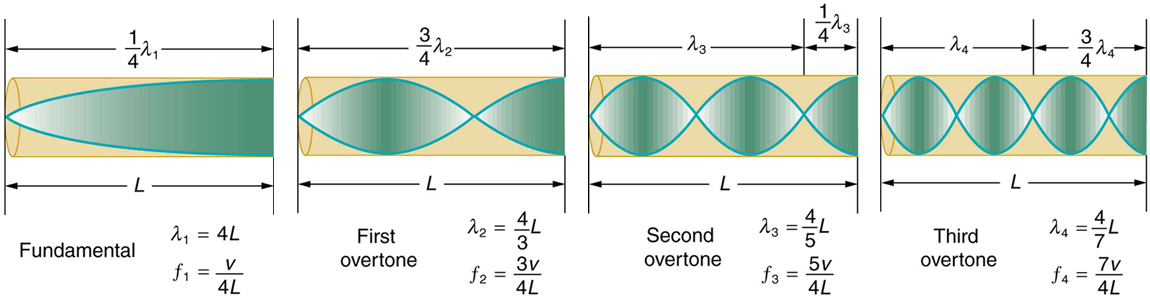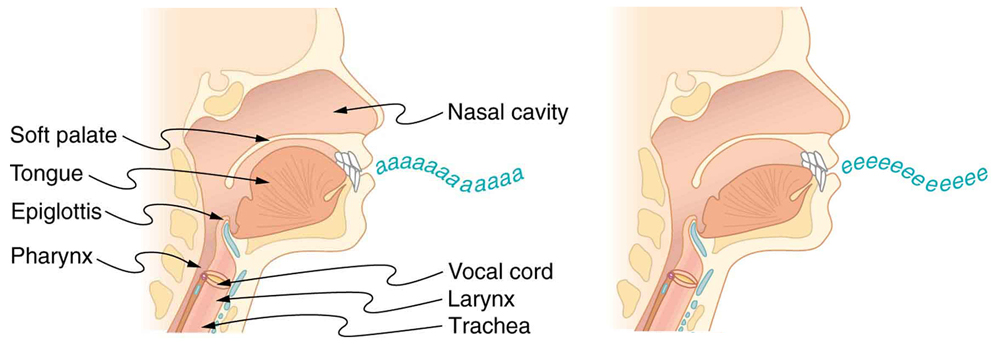# 17.5 Sound interference and resonance: standing waves in air columns  (Page 3/11)

 Page 3 / 11Another resonance for a tube closed at one end. This has maximum air displacements at the open end, and none at the closed end. The wavelength is shorter, with three-fourths λ ′ size 12{ { {λ}} sup { ' }} {} equaling the length of the tube, so that λ ′ = 4 L / 3 size 12{ { {λ}} sup { ' }=4L/3} {} . This higher-frequency vibration is the first overtone.The fundamental and three lowest overtones for a tube closed at one end. All have maximum air displacements at the open end and none at the closed end.

The fundamental and overtones can be present simultaneously in a variety of combinations. For example, middle C on a trumpet has a sound distinctively different from middle C on a clarinet, both instruments being modified versions of a tube closed at one end. The fundamental frequency is the same (and usually the most intense), but the overtones and their mix of intensities are different and subject to shading by the musician. This mix is what gives various musical instruments (and human voices) their distinctive characteristics, whether they have air columns, strings, sounding boxes, or drumheads. In fact, much of our speech is determined by shaping the cavity formed by the throat and mouth and positioning the tongue to adjust the fundamental and combination of overtones. Simple resonant cavities can be made to resonate with the sound of the vowels, for example. (See [link] .) In boys, at puberty, the larynx grows and the shape of the resonant cavity changes giving rise to the difference in predominant frequencies in speech between men and women.The throat and mouth form an air column closed at one end that resonates in response to vibrations in the voice box. The spectrum of overtones and their intensities vary with mouth shaping and tongue position to form different sounds. The voice box can be replaced with a mechanical vibrator, and understandable speech is still possible. Variations in basic shapes make different voices recognizable.

Now let us look for a pattern in the resonant frequencies for a simple tube that is closed at one end. The fundamental has $\lambda =4L$ , and frequency is related to wavelength and the speed of sound as given by:

${v}_{w}=\mathrm{f\lambda .}$

Solving for $f$ in this equation gives

$f=\frac{{v}_{w}}{\lambda }=\frac{{v}_{w}}{4L},$

where ${v}_{w}$ is the speed of sound in air. Similarly, the first overtone has $\lambda \prime =4L/3$ (see [link] ), so that

$f\prime =3\frac{{v}_{w}}{4L}=3f.$

Because $f\prime =3f$ , we call the first overtone the third harmonic. Continuing this process, we see a pattern that can be generalized in a single expression. The resonant frequencies of a tube closed at one end are

${f}_{n}=n\frac{{v}_{w}}{4L}\text{,}\phantom{\rule{0.25em}{0ex}}n=1,3,5\text{,}$

where ${f}_{1}$ is the fundamental, ${f}_{3}$ is the first overtone, and so on. It is interesting that the resonant frequencies depend on the speed of sound and, hence, on temperature. This dependence poses a noticeable problem for organs in old unheated cathedrals, and it is also the reason why musicians commonly bring their wind instruments to room temperature before playing them.

## Find the length of a tube with a 128 hz fundamental

(a) What length should a tube closed at one end have on a day when the air temperature, is $22.0ºC$ , if its fundamental frequency is to be 128 Hz (C below middle C)?

(b) What is the frequency of its fourth overtone?

Strategy

The length $L$ can be found from the relationship in ${f}_{n}=n\frac{{v}_{w}}{4L}$ , but we will first need to find the speed of sound ${v}_{w}$ .

Solution for (a)

(1) Identify knowns:

• the fundamental frequency is 128 Hz
• the air temperature is $22.0ºC$

(2) Use ${f}_{n}=n\frac{{v}_{w}}{4L}$ to find the fundamental frequency ( $n=1$ ).

${f}_{1}=\frac{{v}_{w}}{4L}$

(3) Solve this equation for length.

$L=\frac{{v}_{w}}{{4f}_{1}}$

(4) Find the speed of sound using ${v}_{w}=\left(\text{331 m/s}\right)\sqrt{\frac{T}{\text{273 K}}}$ .

${v}_{w}=\left(\text{331 m/s}\right)\sqrt{\frac{\text{295 K}}{\text{273 K}}}=\text{344 m/s}$

(5) Enter the values of the speed of sound and frequency into the expression for $L$ .

$L=\frac{{v}_{w}}{{4f}_{1}}=\frac{\text{344 m/s}}{4\left(\text{128 Hz}\right)}=0\text{.}\text{672 m}$

Discussion on (a)

Many wind instruments are modified tubes that have finger holes, valves, and other devices for changing the length of the resonating air column and hence, the frequency of the note played. Horns producing very low frequencies, such as tubas, require tubes so long that they are coiled into loops.

Solution for (b)

(1) Identify knowns:

• the first overtone has $n=3$
• the second overtone has $n=5$
• the third overtone has $n=7$
• the fourth overtone has $n=9$

(2) Enter the value for the fourth overtone into ${f}_{n}=n\frac{{v}_{w}}{4L}$ .

${f}_{9}=9\frac{{v}_{w}}{4L}={9f}_{1}=\text{1.15 kHz}$

Discussion on (b)

Whether this overtone occurs in a simple tube or a musical instrument depends on how it is stimulated to vibrate and the details of its shape. The trombone, for example, does not produce its fundamental frequency and only makes overtones.

What is the difference between a principle and a law
the law is universally proved. The principal depends on certain conditions.
Dr
what does the speedometer of a car measure ?
Car speedometer measures the rate of change of distance per unit time.
Moses
describe how a Michelson interferometer can be used to measure the index of refraction of a gas (including air)
using the law of reflection explain how powder takes the shine off a person's nose. what is the name of the optical effect?
WILLIAM
is higher resolution of microscope using red or blue light?.explain
WILLIAM
can sound wave in air be polarized?
Unlike transverse waves such as electromagnetic waves, longitudinal waves such as sound waves cannot be polarized. ... Since sound waves vibrate along their direction of propagation, they cannot be polarized
Astronomy
A proton moves at 7.50×107m/s perpendicular to a magnetic field. The field causes the proton to travel in a circular path of radius 0.800 m. What is the field strength?
derived dimenionsal formula
what is the difference between mass and weight
assume that a boy was born when his father was eighteen years.if the boy is thirteen years old now, how is his father in
Isru
what is airflow
derivative of first differential equation
why static friction is greater than Kinetic friction
draw magnetic field pattern for two wire carrying current in the same direction
An American traveler in New Zealand carries a transformer to convert New Zealand’s standard 240 V to 120 V so that she can use some small appliances on her trip.
What is the ratio of turns in the primary and secondary coils of her transformer?
nkombo
what is energy
Yusuf
How electric lines and equipotential surface are mutually perpendicular?
The potential difference between any two points on the surface is zero that implies È.Ŕ=0, Where R is the distance between two different points &E= Electric field intensity. From which we have cos þ =0, where þ is the angle between the directions of field and distance line, as E andR are zero. Thus
sorry..E and R are non zero...

#### Get Jobilize Job Search Mobile App in your pocket Now!ByByBy JavaChamp TeamBy OpenStaxBy OpenStaxBy OpenStaxBy Ann SchlosserBy Charles JumperBy Sandhills MLTBy Christine ZeelieBy Madison ChristianBy Subramanian Divya